# MA.912.AR.3.1Export Print
Given a mathematical or real-world context, write and solve one-variable quadratic equations over the real number system.

### Clarifications

Clarification 1: Within the Algebra 1 course, instruction includes the concept of non-real answers, without determining non-real solutions.

Clarification 2: Within this benchmark, the expectation is to solve by factoring techniques, taking square roots, the quadratic formula and completing the square.

General Information
Subject Area: Mathematics (B.E.S.T.)
Strand: Algebraic Reasoning
Status: State Board Approved

## Benchmark Instructional Guide

### Terms from the K-12 Glossary

• Coefficient
• Real Numbers
• $x$-intercept

### Vertical Alignment

Previous Benchmarks

Next Benchmarks

### Purpose and Instructional Strategies

In grade 8, students solved quadratic equations in the form of $x$2 = $p$. In Algebra I, students solve quadratic equations in one variable over the real number system. In later courses, students will solve quadratic equations in one variable over the real and complex number systems.
• Instruction includes the use of manipulatives, models, drawings and various methods, including Loh’s method.
• Instruction allows the flexibility to solve quadratics using factoring techniques, taking the square root, using the quadratic formula and completing the square. Students should understand that one method may be more efficient than another depending on the content and context of the problem (MTR.2.1).
• Instruction emphasizes the understanding that solving a quadratic equation in one variable is the same as the process of determining $x$-intercepts, or roots, of the graph of a quadratic function.
• While the derivation of the quadratic formula is not an expectation of this benchmark, students can develop the quadratic formula by using completing the square to isolate $x$ in the equation $a$$x$2 + $b$$x$ + $c$ = 0; making the connection to solving literal equations.
• Instruction includes evaluating the discriminant ($b$2– 4$a$$c$) to determine whether there is one real solution (equals zero), two real solutions (equals a positive rational number) or two complex solutions (equals a negative rational number).
• Discuss the connection of the number of solutions of a quadratic equation to the graph of a quadratic function. Guide students to see that real solutions result in roots or $x$-intercepts and that quadratic functions that do not produce real roots never touch the $x$-axis (MTR.5.1).
• Instruction on completing the square includes the use of algebra tiles or area model drawings. Students should understand the visual nature of completing the square and connect it to their prior work with area models.
• For example, consider $x$2 + 8$x$ = 48 and solve by factoring to show that the two solutions are $x$ = −12 and $x$ = 4. Then, ask the question “If we look at the binomial $x$2 + 8$x$ on the left, what would we have to add to it to make a perfect square trinomial?” Write the equation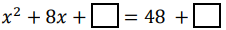on the board to represent the question. Now, represent the equation using algebra tiles or by drawing an area model as shown below.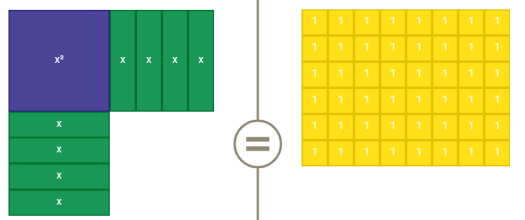• Lead student discussion about the quantity of 1 unit by 1 unit tiles needed to “complete the square” on the left hand side. Once they state the need for 16 tiles, point out that 16 tiles must also be added to the right to maintain equivalence then write the number 16 into both blank boxes. Students can then factor the perfect square trinomial to arrive at ($x$ + 4)² = 64. Have student solve using square roots to find the same solutions of $x$ = −12 and $x$ = 4 (MTR.2.1MTR.4.1MTR.5.1).
• In many contexts, students may generate solutions that may not make sense when placed in context. Be sure students assess the reasonableness of their solutions in terms of context to check for this (MTR.6.1).
• For example, the time it takes for a ball to drop from a height of 28 feet can be modeled by 0 = −16$t$2 + 28. Students solve this equation to find that $t$ ≈ ±1.32 seconds. Through discussion, students should see that −1.32 seconds does not make sense in context and therefore should be omitted.
• Enrichment of this benchmark includes determining if a quadratic is a perfect square trinomial.
• For example, given the equation 0 = 4$x$2 − 12$x$ + 9, students can identify $a$, $b$, and $c$ as 4, −12 and 9, respectively. Students should recognize that $a$ and $c$ are perfect squares; where $a$ is 22= 4 and $c$ is (−3)= 9. Since the coefficient of $b$ is twice the product of the square roots (−12 = 2(2)(−3)), it can be determined that the given equation is a perfect square trinomial.

### Common Misconceptions or Errors

• When completing the square, many students forget to use the addition or subtraction property of equality to add or subtract values from the other side of the equation. Remind these students that additions or subtractions from one side of an equation must be replicated on the other to maintain equivalency.
• When completing the square and removing a common factor from the $x$2 and $x$ term, students may forget to consider that factor when adding/subtracting from the other side of the equation.
• For example, when solving 10 = 12$t$2 + $t$ + 55, students may ultimately add 4 rather than 48 to the left side. Help students to see that there are 12 sets of 4 ultimately being added to the right and therefore, there must be twelve sets added to the left as well.
• Some students may see equations such as $x$2 – 4$x$ + 6 = 27 and use the number 2, −4 and 6 as $a$, $b$ and $c$, respectively, in the quadratic formula, arriving at an incorrect solutions. In these cases, graph the related function and ask students if the solutions they calculated correspond to the roots of the parabola. Once they see they do not, have students set the equation equal to zero and recalculate.

### Strategies to Support Tiered Instruction

• When solving using the quadratic formula, instruction includes separating the two possible solutions to two equations.
• For example, when determining the values of $x$ in the equation 6$x$2 − 17$x$ + 12 = 0, students can set up the two equations using the quadratic formula: $x$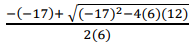and $x$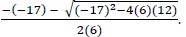• Teacher provides guided notes that show step-by-step directions for solving problems.
• For example, teacher can use the directions below when using the quadratic formula.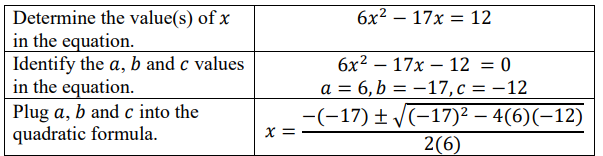• Teacher co-creates a graphic organizer, such as the one below, to include different methods used to solve quadratics given specific forms.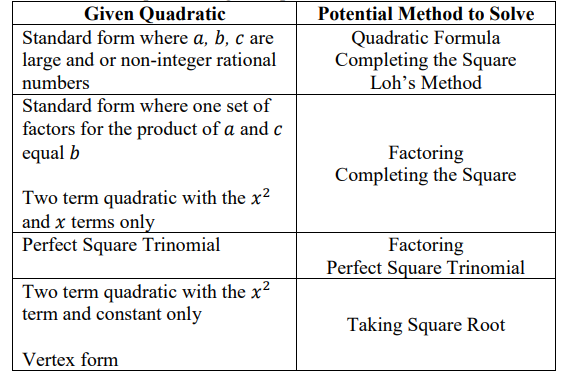• Given the equation $x$2 + 6$x$ = 13, what value(s) of $x$ satisfy the equation?

• Given the figure below, write an equation that could be used to determine the length and width of the rectangle.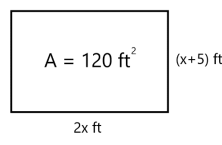### Instructional Items

Instructional Item 1
• Devonte throws a rock straight down off the edge of a cliff that overlooks the ocean. The distance ($d$) the rock falls after t seconds can be represented by the equation $d$ = 16$t$2+ 24$t$. If the ocean’s surface is 16.4 feet below the cliff, to the nearest tenth, how many seconds will it take for the rock to hit the ocean’s surface?

Instructional Item 2
•  What are the solutions to the equation −0.25$x$2 + 4$x$ = 0.75. Round to the nearest tenth if necessary.

Instructional Item 3
• What are the exact solutions to the equation 5$x$$\frac{\text{17}}{\text{2}}$$x$ + $\frac{\text{3}}{\text{2}}$ = 0?

*The strategies, tasks and items included in the B1G-M are examples and should not be considered comprehensive.

## Related Courses

This benchmark is part of these courses.
1200310: Algebra 1 (Specifically in versions: 2014 - 2015, 2015 - 2022, 2022 and beyond (current))
1200320: Algebra 1 Honors (Specifically in versions: 2014 - 2015, 2015 - 2022, 2022 and beyond (current))
1200380: Algebra 1-B (Specifically in versions: 2014 - 2015, 2015 - 2022, 2022 and beyond (current))
1200400: Foundational Skills in Mathematics 9-12 (Specifically in versions: 2014 - 2015, 2015 - 2022, 2022 and beyond (current))
7912090: Access Algebra 1B (Specifically in versions: 2014 - 2015, 2015 - 2018, 2018 - 2019, 2019 - 2022, 2022 and beyond (current))
1200315: Algebra 1 for Credit Recovery (Specifically in versions: 2014 - 2015, 2015 - 2022, 2022 and beyond (current))
1200385: Algebra 1-B for Credit Recovery (Specifically in versions: 2014 - 2015, 2015 - 2022, 2022 and beyond (current))
7912075: Access Algebra 1 (Specifically in versions: 2014 - 2015, 2015 - 2018, 2018 - 2019, 2019 - 2022, 2022 and beyond (current))
1209315: Mathematics for ACT and SAT (Specifically in versions: 2022 and beyond (current))

## Related Access Points

Alternate version of this benchmark for students with significant cognitive disabilities.
MA.912.AR.3.AP.1: Given a one-variable quadratic equation from a mathematical or real-world context, select the solution to the equation over the real number system.

## Related Resources

Vetted resources educators can use to teach the concepts and skills in this benchmark.

## Formative Assessments

Quilts:

Students are asked to write and solve an equation that models a given problem.

Type: Formative Assessment

Complete the Square - 1:

Students are asked to solve a quadratic equation by completing the square.

Type: Formative Assessment

Complete the Square - 2:

Students are asked to solve a quadratic equation by completing the square.

Type: Formative Assessment

Complete the Square - 3:

Students are asked to solve a quadratic equation by completing the square.

Type: Formative Assessment

Which Strategy?:

Students are shown four quadratic equations and asked to choose the best method for solving each equation.

Type: Formative Assessment

## Lesson Plans

Solving Quadratics - Exploring Different Methods:

Students will explore how different methods find the solutions (roots) to quadratic equations including, factoring, graphing, and the quadratic formula.

Type: Lesson Plan

Hip to be (completing the) Square:

This lesson is an introduction to completing the square. Students will learn what it means to "complete the square" with a quadratic trinomial expression. They will practice both with manipulatives and mathematically, and will then use that information to find the maximum or minimum value of an expression using the vertex form of a quadratic. This lesson moves through all levels of Concrete, Representational, Abstract instruction.

Type: Lesson Plan

Students will sort various quadratic equations by the method they would use for solving (ie. factoring, quadratic formula). Then as a class they justify their placements and eventually discover that there are many ways to solve and that some make sense in different situations, however there is no real "correct" method for each equation type.

Type: Lesson Plan

## Original Student Tutorials

Highs and Lows Part 2: Completing the Square:

Learn the process of completing the square of a quadratic function to find the maximum or minimum to discover how high a dolphin jumped in this interactive tutorial.

This is part 2 of a 2 part series. Click HERE to open part 1.

Type: Original Student Tutorial

Highs and Lows Part 1: Completing the Square:

Learn the process of completing the square of a quadratic function to find the maximum or minimum to discover how high a dolphin jumped in this interactive tutorial.

This is part 1 of a 2 part series. Click HERE to open Part 2.

Type: Original Student Tutorial

Solving Rational Equations: Cross Multiplying:

Learn how to solve rational linear and quadratic equations using cross multiplication in this interactive tutorial.

Type: Original Student Tutorial

## MFAS Formative Assessments

Complete the Square - 1:

Students are asked to solve a quadratic equation by completing the square.

Complete the Square - 2:

Students are asked to solve a quadratic equation by completing the square.

Complete the Square - 3:

Students are asked to solve a quadratic equation by completing the square.

Quilts:

Students are asked to write and solve an equation that models a given problem.

Which Strategy?:

Students are shown four quadratic equations and asked to choose the best method for solving each equation.

## Original Student Tutorials Mathematics - Grades 9-12

Highs and Lows Part 1: Completing the Square:

Learn the process of completing the square of a quadratic function to find the maximum or minimum to discover how high a dolphin jumped in this interactive tutorial.

This is part 1 of a 2 part series. Click HERE to open Part 2.

Highs and Lows Part 2: Completing the Square:

Learn the process of completing the square of a quadratic function to find the maximum or minimum to discover how high a dolphin jumped in this interactive tutorial.

This is part 2 of a 2 part series. Click HERE to open part 1.

Solving Rational Equations: Cross Multiplying:

Learn how to solve rational linear and quadratic equations using cross multiplication in this interactive tutorial.

## Student Resources

Vetted resources students can use to learn the concepts and skills in this benchmark.

## Original Student Tutorials

Highs and Lows Part 2: Completing the Square:

Learn the process of completing the square of a quadratic function to find the maximum or minimum to discover how high a dolphin jumped in this interactive tutorial.

This is part 2 of a 2 part series. Click HERE to open part 1.

Type: Original Student Tutorial

Highs and Lows Part 1: Completing the Square:

Learn the process of completing the square of a quadratic function to find the maximum or minimum to discover how high a dolphin jumped in this interactive tutorial.

This is part 1 of a 2 part series. Click HERE to open Part 2.

Type: Original Student Tutorial

Solving Rational Equations: Cross Multiplying:

Learn how to solve rational linear and quadratic equations using cross multiplication in this interactive tutorial.

Type: Original Student Tutorial

## Parent Resources

Vetted resources caregivers can use to help students learn the concepts and skills in this benchmark.